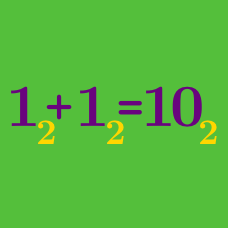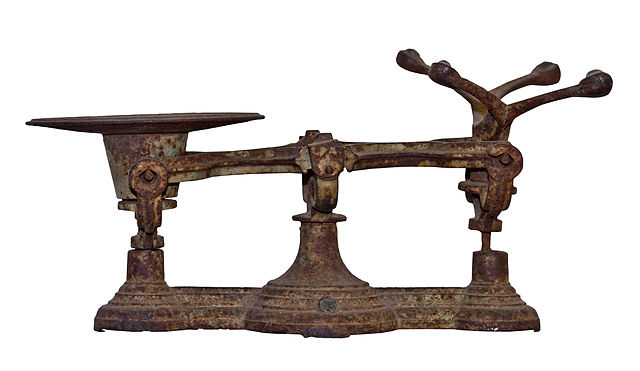Number Theory

# Number Bases: Level 4 Challenges

Let $N$ be the number that consists of 61 consecutive 3's, so $N = \underbrace{333\ldots333}_{61 \, 3's}$. Let $M$ be the number that consists of 62 consecutive 6's, so $M=\underbrace{6666\ldots666}_{62 \, 6's}$. What is the digit sum of $N\times M$?

Details and assumptions

The digit sum of a number is the sum of all its digits. For example the digit sum of 1123 is $1 + 1 + 2 + 3 = 7$.

Let $f$ be a polynomial with nonnegative integer coefficients. If $f(1)=7$ and $f(7)=7597$, what is $f(10)?$You have a weighing balance, which you can place weights on both sides of. You need to measure all weights between 1 and 1000.

For example if you have weights 1 and 3, you can measure test objects of weights 1, 3 or 4. You can also measure objects of weight 2, by placing 3 on one side and 1 on the side which contain the object to be weighed.

What is the minimum number of weights that you would need to be able to measure all (integral) weights from 1 kg to 1000 kg?

Calvin went to a newly discovered planet called "Pandora" to research their advancements in the field of Mathematics. He found the following equation scrawled in the dust:

$\large{3x^2 - 25x + 66 = 0 \quad \Longrightarrow \quad x=4 \text{ or } x=9}$

Can you help Calvin in finding the base, which is used in the Number System on Pandora?

Note: Symbols for digits in the Pandora System and Decimal system have the same value. e.g. 6 in Pandora represents six.

Find the number of integers that are between $1$ and $100$ inclusive that when converted to binary have a digit sum of less than $5.$

×